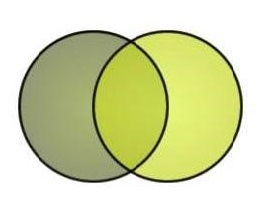# imaginary numbers

n=6

a: imaginary numbers ~

What:

"I like when the metaphors are more complicated than the referent. Here's my answer: imaginary numbers are like your crazy uncle...there's internal consistency to the things he's mumbling, but it may not make much sense to outsiders."

Useful?
Writer: Maserschmidt
LCC:
Where:
Date: Aug 21 2014 4:39 PM

a: imaginary numbers ~
b: mathematical scaffolding

What:

"I am starting to take the view that imaginary numbers are like mathematical scaffolding, they help get to the next level, but not quite strong enough to build a lasting foundation."

Useful?
Writer: Not Stated
LCC:
Where:
Date: Aug 21 2014 4:42 PM

a: Imaginary numbers ~
b: spirits

What:

"Imaginary numbers are like spirits wandering between being and nothingness, and are again much more numerous than real numbers."

Useful?
Writer: Koji Suzuki
LCC:
Where:
Date: Aug 21 2014 4:46 PM

a: imaginary numbers ~
b: the Holy Ghost

What:

"As another instance of Leibniz' theological simulacrums, we have his remark that imaginary numbers are like the Holy Ghost of Christian scriptures-a sort of amphibian, midway between existence and nonexistence."

Useful?
Writer: Not Stated
LCC:
Where:
Date: Aug 21 2014 5:08 PM

a: Imaginary numbers ~
b: y-axis

What:

"It's more like two-dimensional. Real numbers are like the x-axis on the complex plane, and imaginary numbers are like the y-axis. Another way to look at it is, each real number can be described as itself times the multiplicative unit, i.e. 5 is 5*sqrt(1), 24 is 24*sqrt(1), 1 is sqrt(1)2. That's their 'x unit'. Each imaginary number can be described as a number times the imaginary unit, sqrt(-1), or i. We'd say that the number 5i is imaginary and is equal to 5 times the imaginary unit. In geometric terms, it's 5 y-units on the complex plane. When you mix together imaginary and real numbers, you get a complex number, describing both its coordinates on the complex plane, except instead of (5, 10) for 5x and 10y, we have (5 + 10i) for 5*sqrt(1) and 10*sqrt(-1). I hope this is making some kind of sense. "

Useful?
Writer: Appathy
LCC:
Where:
Date: Aug 21 2014 5:09 PM

a: imaginary numbers ~
b: 90-degree rotation

What:

"Imaginary numbers let us rotate numbers. Don't start by defining i as the square root of -1. Show how if negative numbers represent a 180-degree rotation, imaginary numbers represent a 90-degree one."

Useful?
Writer: Not Stated
LCC:
Where:
Date: Jun 25 2017 12:22 PMMETAMIA is a free database of analogy and metaphor. Anyone can contribute or search. The subject matter can be anything. Science is popular, but poetry is encouraged. The goal is to integrate our fluid muses with the stark literalism of a relational database. Metamia is like a girdle for your muses, a cognitive girdle.